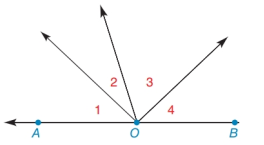Chapter 1.6, Problem 29E### Elementary Geometry for College St...

6th Edition
Daniel C. Alexander + 1 other
ISBN: 9781285195698

#### Solutions

Chapter
Section### Elementary Geometry for College St...

6th Edition
Daniel C. Alexander + 1 other
ISBN: 9781285195698
Textbook Problem
3 views

# Without writing a proof, explain the conclusion for the following problem. Given: A B ↔ Prove: m ∠ 1 + m ∠ 2 + m ∠ 3 + m ∠ 4 = 180To determine

To explain:

The conclusion for the given problem.

Explanation

Given:

The given figure is,

The given line is AB and m1+m2+m3+m4=180°.

Approach:

The sum of angles formed on straight line is supplementary angle. Measure of supplementary angle is 180°.

Calculation:

The angles 1, 2, 3

### Still sussing out bartleby?

Check out a sample textbook solution.

See a sample solution

#### The Solution to Your Study Problems

Bartleby provides explanations to thousands of textbook problems written by our experts, many with advanced degrees!

Get Started

#### Find the limit or show that it does not exist. limx3x22x+1

Single Variable Calculus: Early Transcendentals, Volume I

#### Differentiate. g(x)=1+2x34x

Single Variable Calculus: Early Transcendentals

#### j × (−k) = i −i j + k −j − k

Study Guide for Stewart's Multivariable Calculus, 8th

#### True or False: is a geometric series.

Study Guide for Stewart's Single Variable Calculus: Early Transcendentals, 8th